# MSBSHSE Solutions For Class 8 Maths Part 2 Chapter 12 Equations in one variable

MSBSHSE Solutions For Class 8 Maths Part 2 Chapter 12 Equations in one variable are readily available here. This chapter majorly deals with finding the solution of equations in one variable. The subject matter experts at BYJU’S have outlined all the concepts of this chapter in a clear and precise manner to meet the needs of all students. The solution module provides numerous shortcut tips and tricks to explain all the exercise problems in a simple and easily understandable language. For students who wish to secure high marks, using Maharashtra Board Solutions for Class 8 while solving problems is mandatory.

Here, the students will also learn various other techniques to solve problems pertaining to all the concepts. Further, these solutions are detailed in a step-by-step manner for effective understanding of the problem. Students can find the solutions PDF of Maharashtra State Board Class 8 Textbook Part 2 Chapter 12 Equations in one variable, from the link provided below.

## Download the PDF of Maharashtra Board Solutions For Class 8 Maths Part 2 Chapter 12 Equations in one variable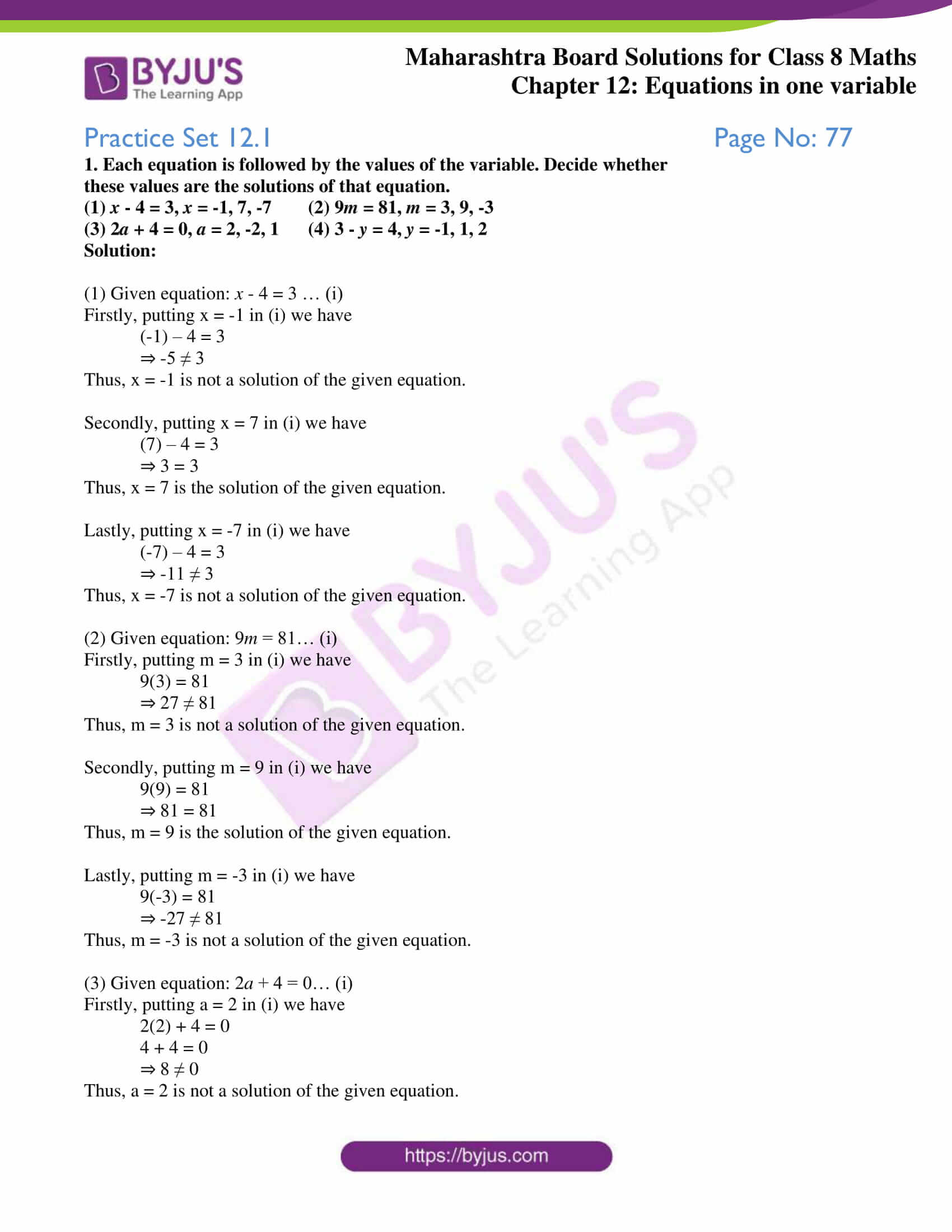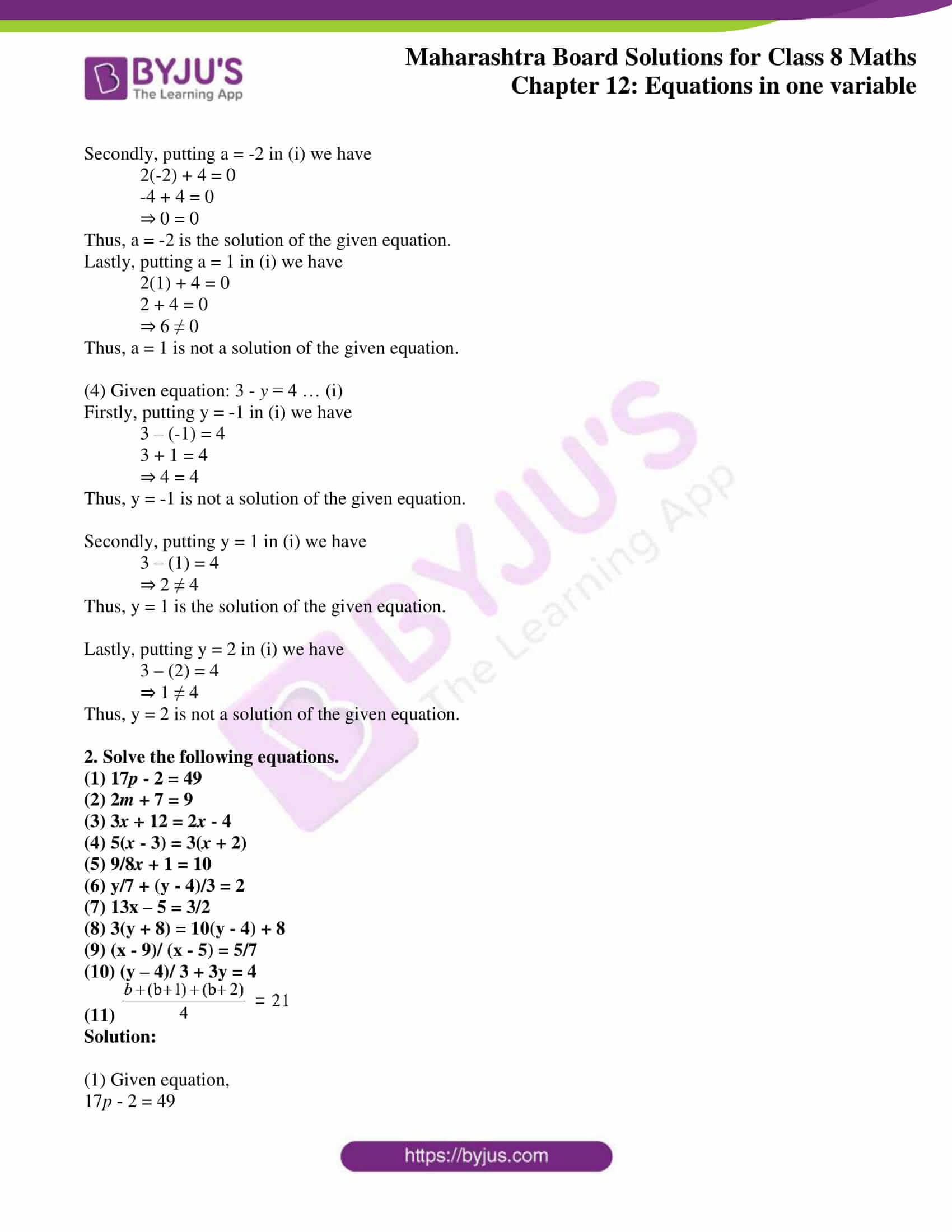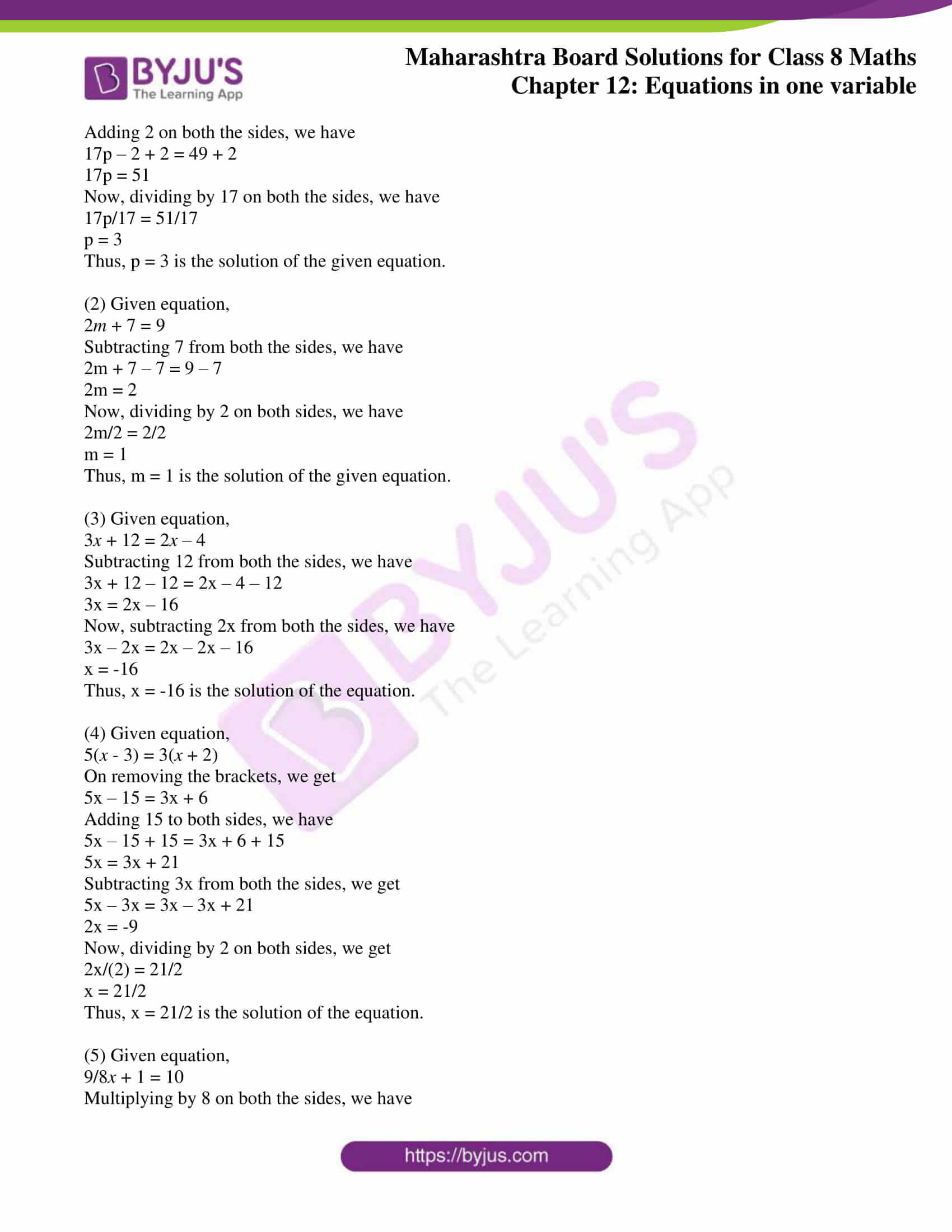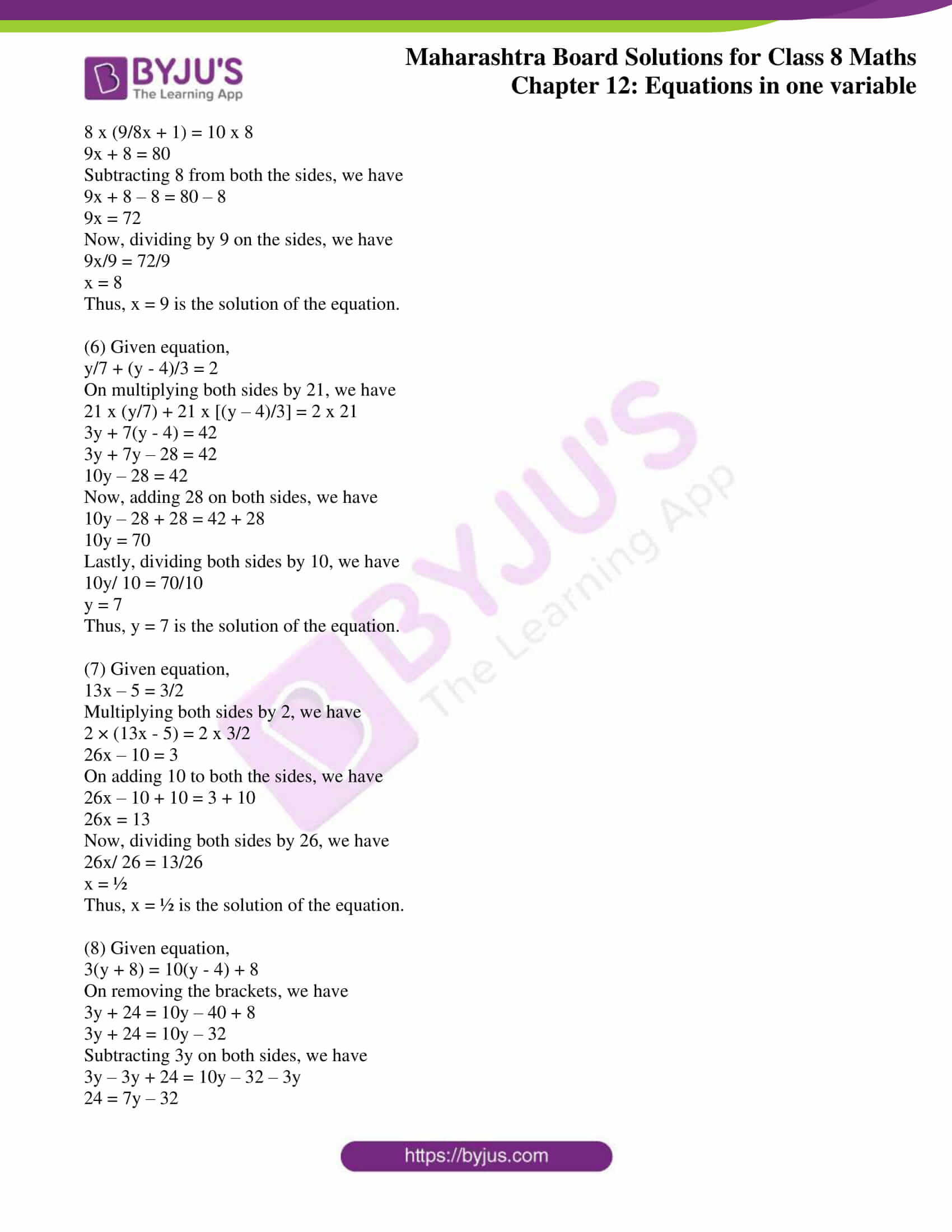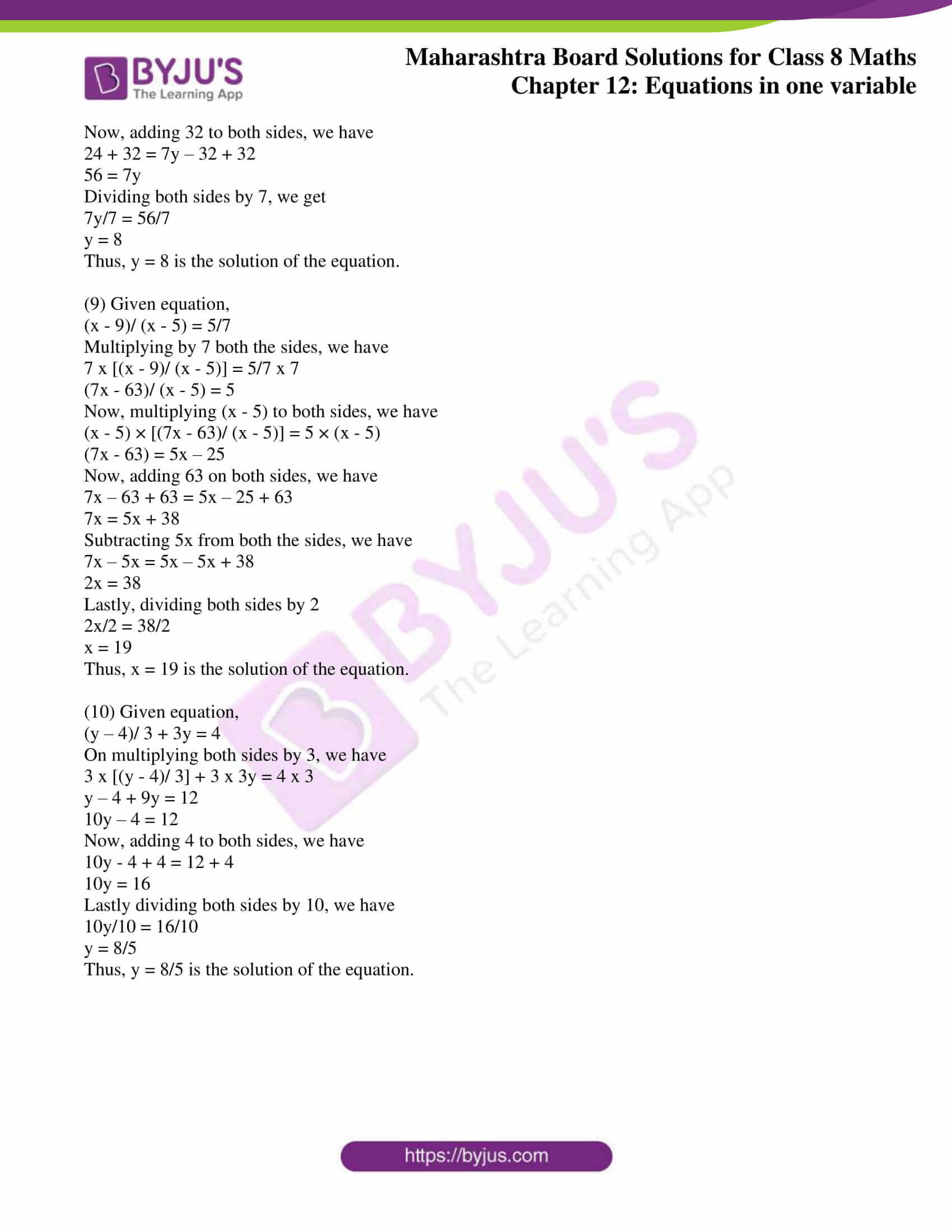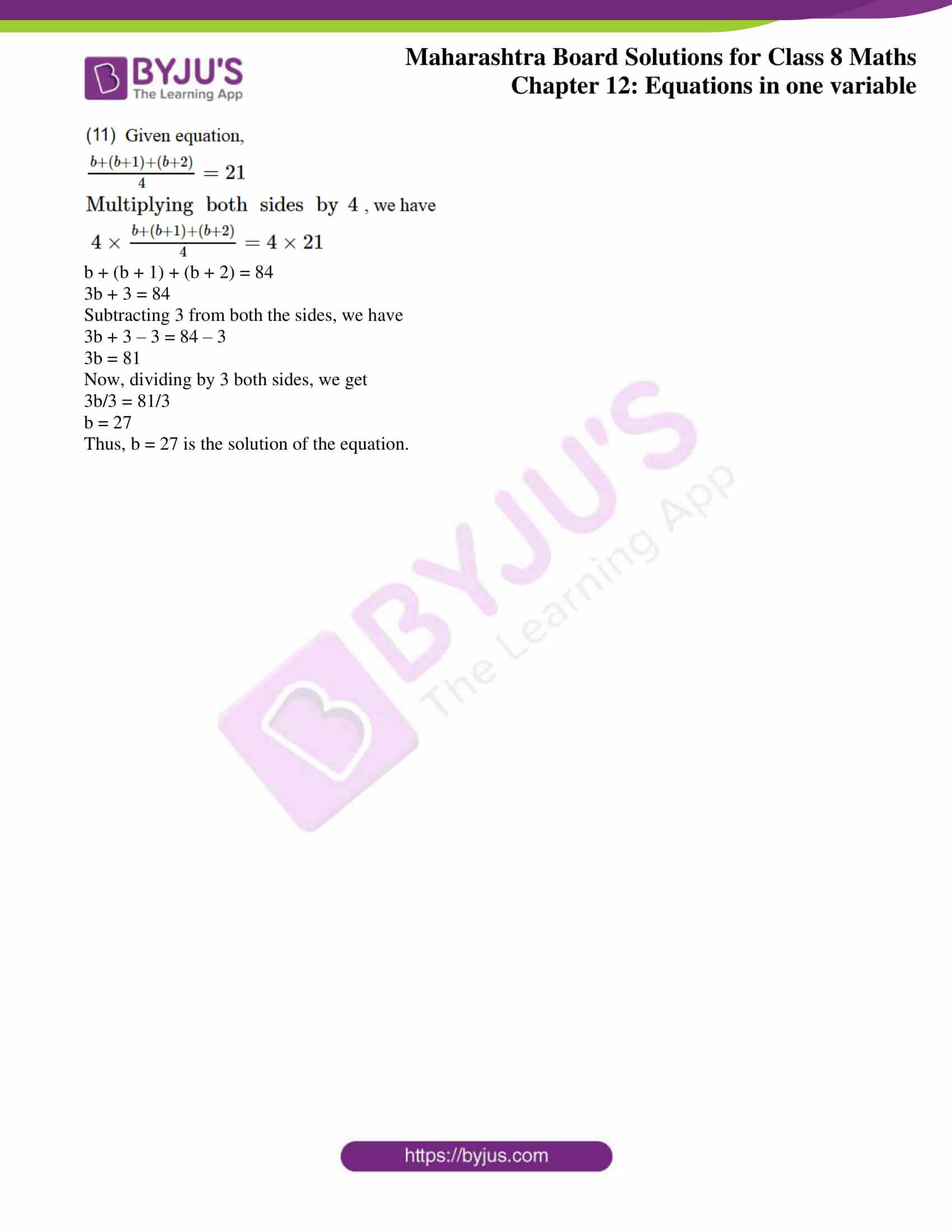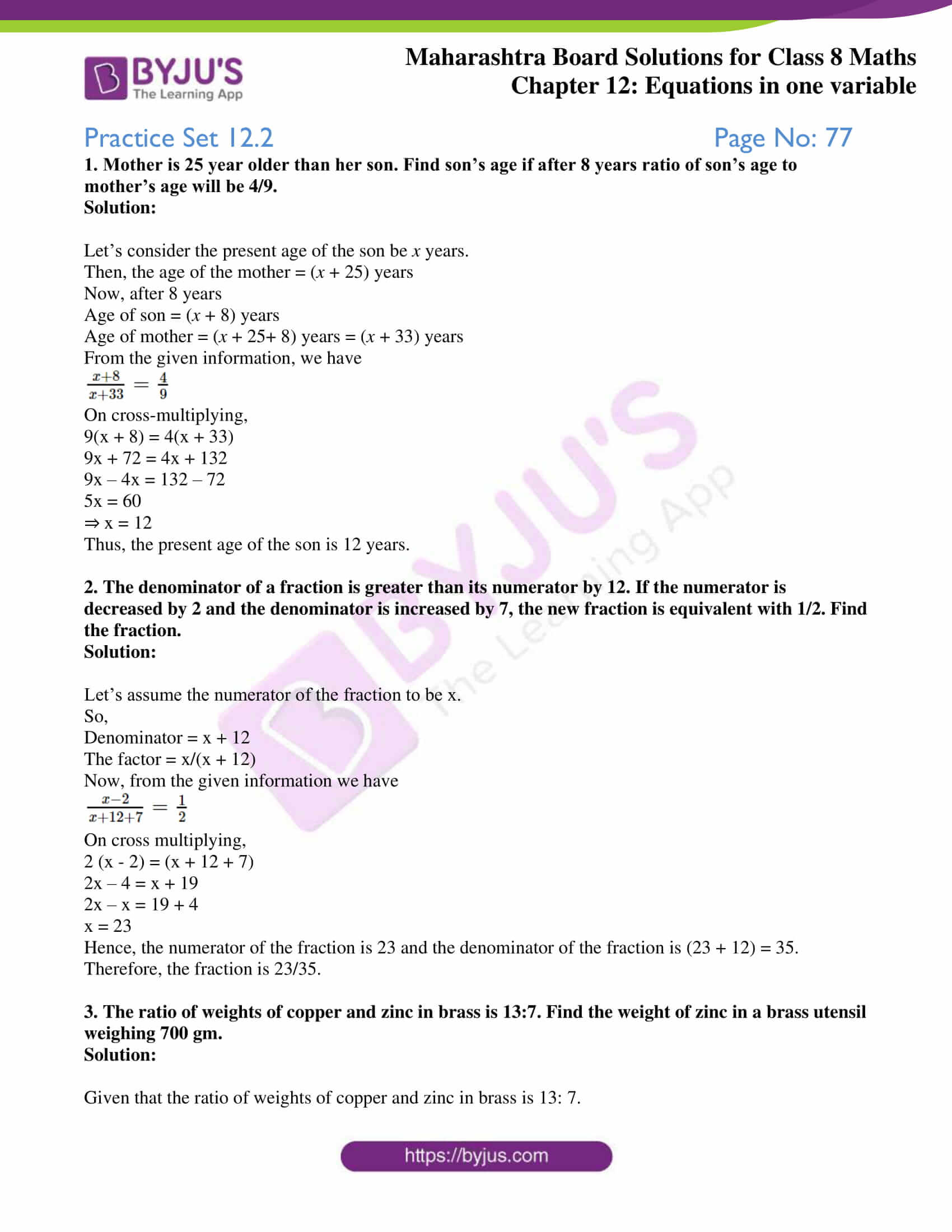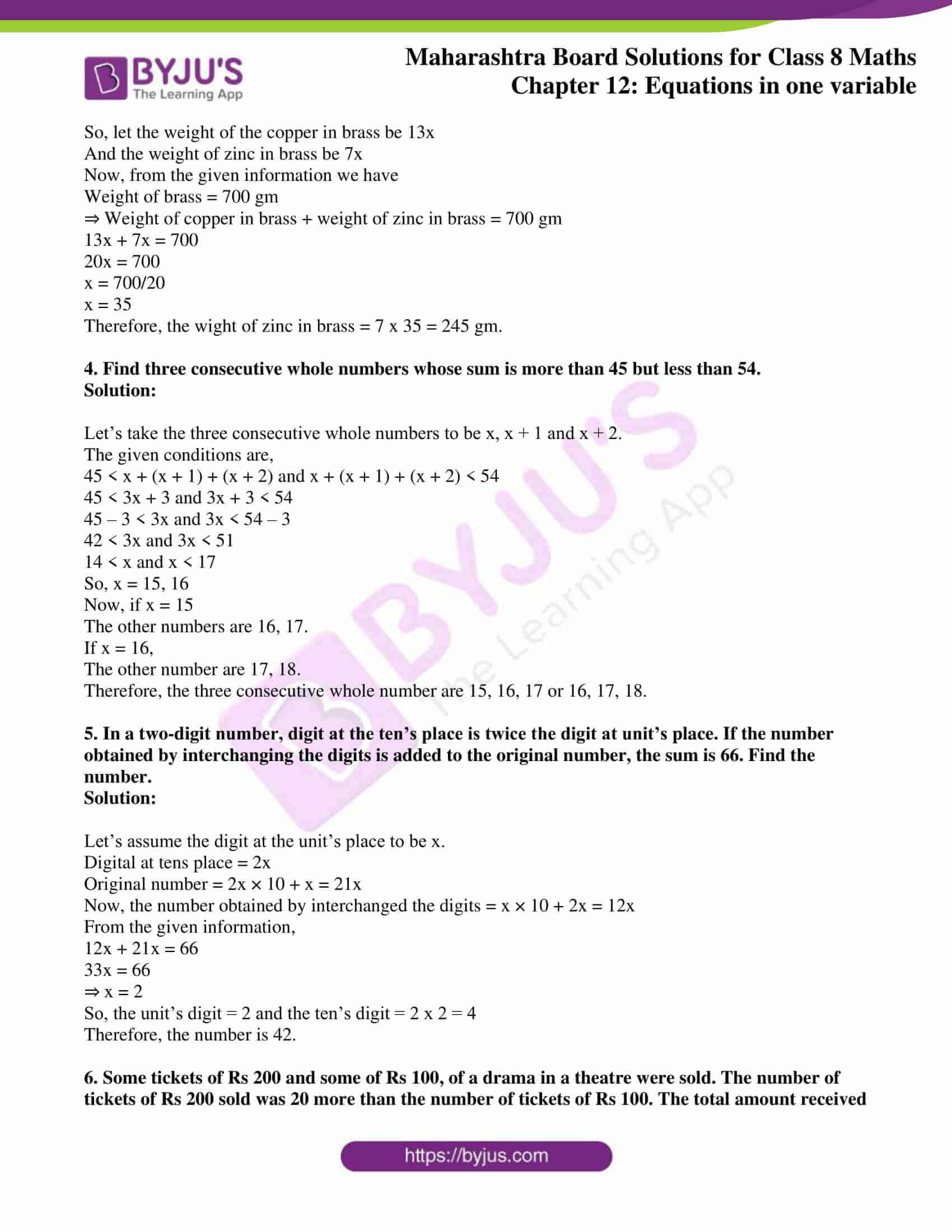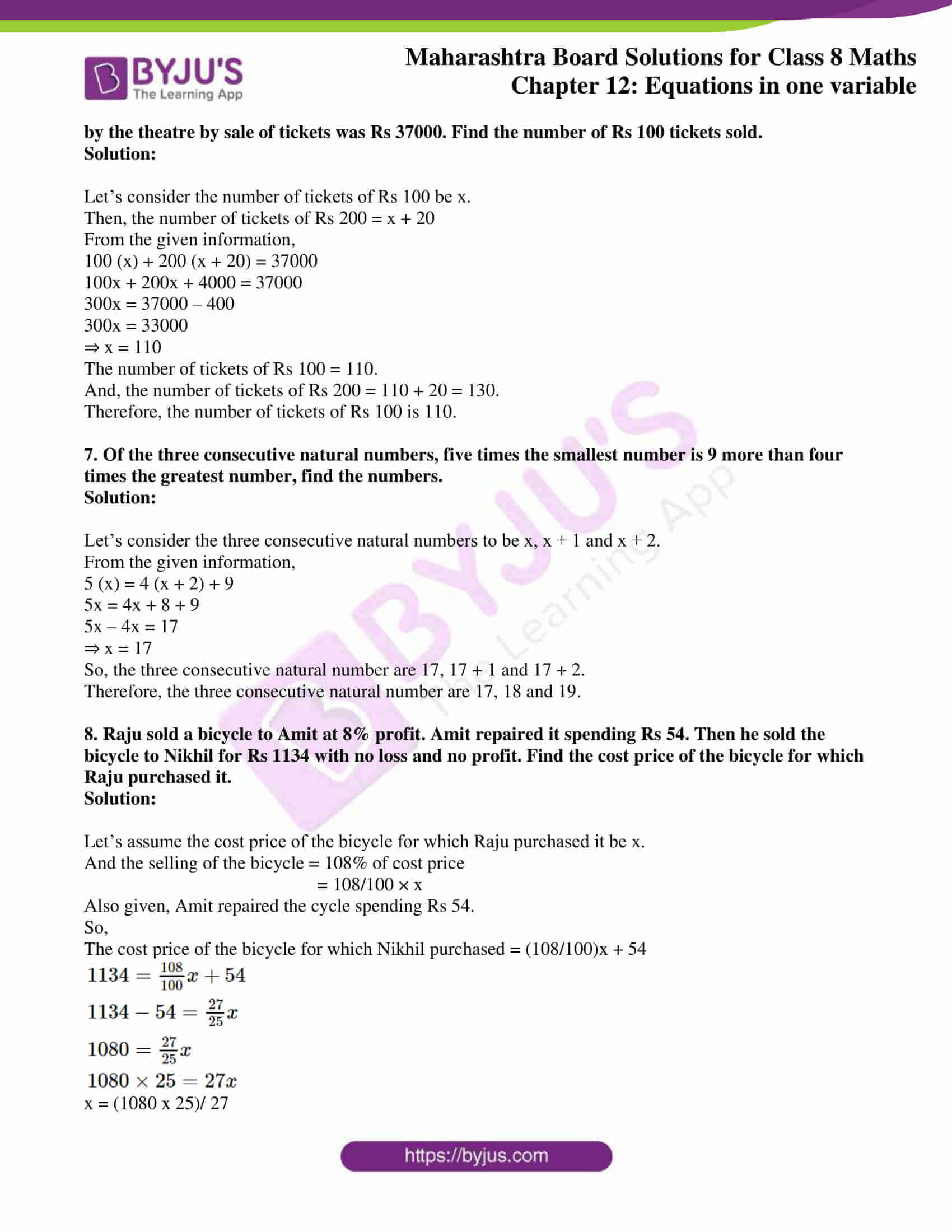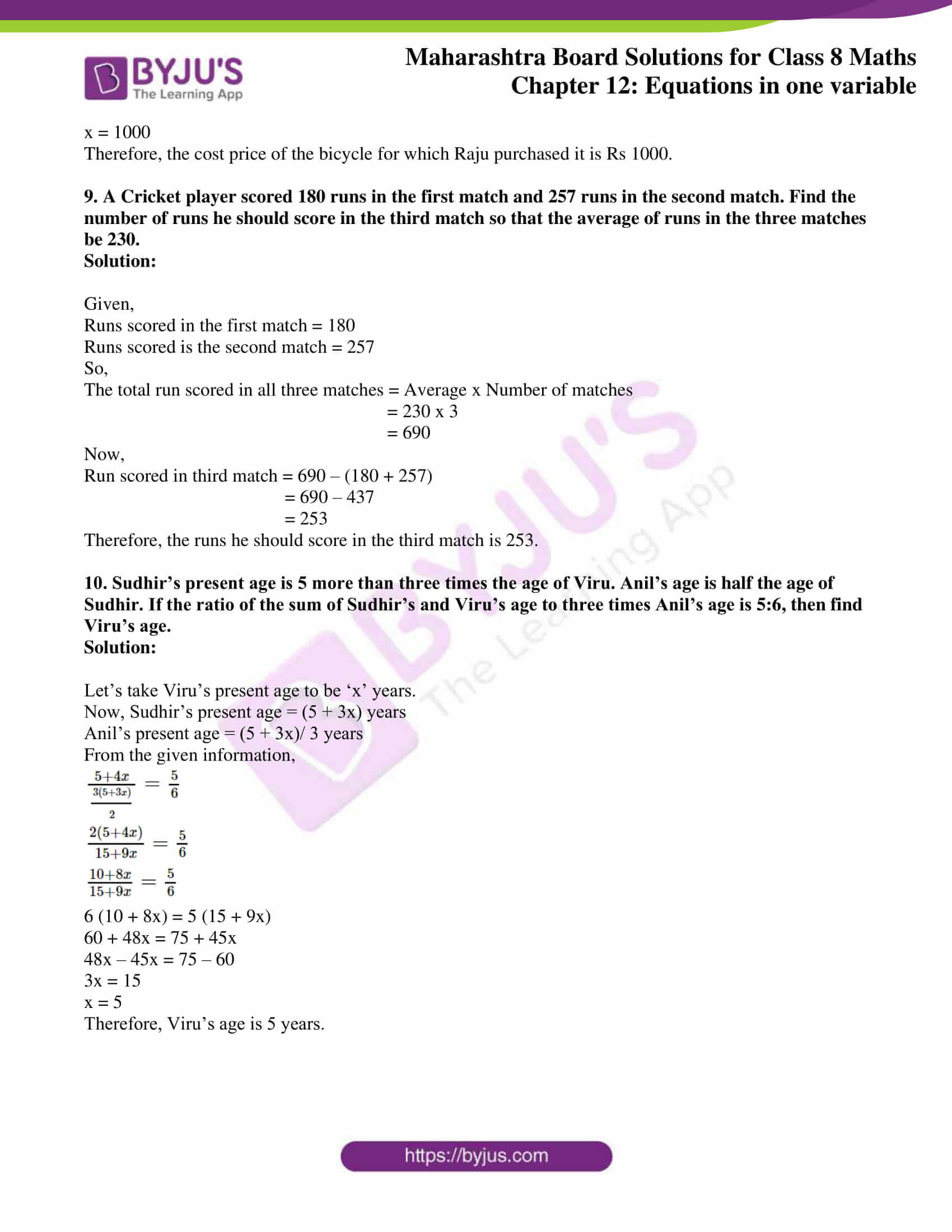### Access answers to Maharashtra Board Solutions For Class 8 Maths Part 2 Chapter 12 Equations in one variable

Practice Set 12.1 Page No: 77

1. Each equation is followed by the values of the variable. Decide whether

these values are the solutions of that equation.

(1) x – 4 = 3, x = -1, 7, -7 (2) 9m = 81, m = 3, 9, -3

(3) 2a + 4 = 0, a = 2, -2, 1 (4) 3 – y = 4, y = -1, 1, 2

Solution:

(1) Given equation: x – 4 = 3 … (i)

Firstly, putting x = -1 in (i) we have

(-1) – 4 = 3

⇒ -5 ≠ 3

Thus, x = -1 is not a solution of the given equation.

Secondly, putting x = 7 in (i) we have

(7) – 4 = 3

⇒ 3 = 3

Thus, x = 7 is the solution of the given equation.

Lastly, putting x = -7 in (i) we have

(-7) – 4 = 3

⇒ -11 ≠ 3

Thus, x = -7 is not a solution of the given equation.

(2) Given equation: 9m = 81… (i)

Firstly, putting m = 3 in (i) we have

9(3) = 81

⇒ 27 ≠ 81

Thus, m = 3 is not a solution of the given equation.

Secondly, putting m = 9 in (i) we have

9(9) = 81

⇒ 81 = 81

Thus, m = 9 is the solution of the given equation.

Lastly, putting m = -3 in (i) we have

9(-3) = 81

⇒ -27 ≠ 81

Thus, m = -3 is not a solution of the given equation.

(3) Given equation: 2a + 4 = 0… (i)

Firstly, putting a = 2 in (i) we have

2(2) + 4 = 0

4 + 4 = 0

⇒ 8 ≠ 0

Thus, a = 2 is not a solution of the given equation.

Secondly, putting a = -2 in (i) we have

2(-2) + 4 = 0

-4 + 4 = 0

⇒ 0 = 0

Thus, a = -2 is the solution of the given equation.

Lastly, putting a = 1 in (i) we have

2(1) + 4 = 0

2 + 4 = 0

⇒ 6 ≠ 0

Thus, a = 1 is not a solution of the given equation.

(4) Given equation: 3 – y = 4 … (i)

Firstly, putting y = -1 in (i) we have

3 – (-1) = 4

3 + 1 = 4

⇒ 4 = 4

Thus, y = -1 is not a solution of the given equation.

Secondly, putting y = 1 in (i) we have

3 – (1) = 4

⇒ 2 ≠ 4

Thus, y = 1 is the solution of the given equation.

Lastly, putting y = 2 in (i) we have

3 – (2) = 4

⇒ 1 ≠ 4

Thus, y = 2 is not a solution of the given equation.

2. Solve the following equations.

(1) 17p – 2 = 49

(2) 2m + 7 = 9

(3) 3x + 12 = 2x – 4

(4) 5(x – 3) = 3(x + 2)

(5) 9/8x + 1 = 10

(6) y/7 + (y – 4)/3 = 2

(7) 13x – 5 = 3/2

(8) 3(y + 8) = 10(y – 4) + 8

(9)
(x – 9)/ (x – 5) = 5/7

(10) (y – 4)/ 3 + 3y = 4

(11)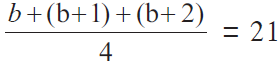Solution:

(1) Given equation,

17p – 2 = 49

Adding 2 on both the sides, we have

17p – 2 + 2 = 49 + 2

17p = 51

Now, dividing by 17 on both the sides, we have

17p/17 = 51/17

p = 3

Thus, p = 3 is the solution of the given equation.

(2) Given equation,

2m + 7 = 9

Subtracting 7 from both the sides, we have

2m + 7 – 7 = 9 – 7

2m = 2

Now, dividing by 2 on both sides, we have

2m/2 = 2/2

m = 1

Thus, m = 1 is the solution of the given equation.

(3) Given equation,

3x + 12 = 2x – 4

Subtracting 12 from both the sides, we have

3x + 12 – 12 = 2x – 4 – 12

3x = 2x – 16

Now, subtracting 2x from both the sides, we have

3x – 2x = 2x – 2x – 16

x = -16

Thus, x = -16 is the solution of the equation.

(4) Given equation,

5(x – 3) = 3(x + 2)

On removing the brackets, we get

5x – 15 = 3x + 6

Adding 15 to both sides, we have

5x – 15 + 15 = 3x + 6 + 15

5x = 3x + 21

Subtracting 3x from both the sides, we get

5x – 3x = 3x – 3x + 21

2x = -9

Now, dividing by 2 on both sides, we get

2x/(2) = 21/2

x = 21/2

Thus, x = 21/2 is the solution of the equation.

(5) Given equation,

9/8x + 1 = 10

Multiplying by 8 on both the sides, we have

8 x (9/8x + 1) = 10 x 8

9x + 8 = 80

Subtracting 8 from both the sides, we have

9x + 8 – 8 = 80 – 8

9x = 72

Now, dividing by 9 on the sides, we have

9x/9 = 72/9

x = 8

Thus, x = 9 is the solution of the equation.

(6) Given equation,

y/7 + (y – 4)/3 = 2

On multiplying both sides by 21, we have

21 x (y/7) + 21 x [(y – 4)/3] = 2 x 21

3y + 7(y – 4) = 42

3y + 7y – 28 = 42

10y – 28 = 42

Now, adding 28 on both sides, we have

10y – 28 + 28 = 42 + 28

10y = 70

Lastly, dividing both sides by 10, we have

10y/ 10 = 70/10

y = 7

Thus, y = 7 is the solution of the equation.

(7) Given equation,

13x – 5 = 3/2

Multiplying both sides by 2, we have

2 × (13x – 5) = 2 x 3/2

26x – 10 = 3

On adding 10 to both the sides, we have

26x – 10 + 10 = 3 + 10

26x = 13

Now, dividing both sides by 26, we have

26x/ 26 = 13/26

x = ½

Thus, x = ½ is the solution of the equation.

(8) Given equation,

3(y + 8) = 10(y – 4) + 8

On removing the brackets, we have

3y + 24 = 10y – 40 + 8

3y + 24 = 10y – 32

Subtracting 3y on both sides, we have

3y – 3y + 24 = 10y – 32 – 3y

24 = 7y – 32

Now, adding 32 to both sides, we have

24 + 32 = 7y – 32 + 32

56 = 7y

Dividing both sides by 7, we get

7y/7 = 56/7

y = 8

Thus, y = 8 is the solution of the equation.

(9) Given equation,

(x – 9)/ (x – 5) = 5/7

Multiplying by 7 both the sides, we have

7 x [(x – 9)/ (x – 5)] = 5/7 x 7

(7x – 63)/ (x – 5) = 5

Now, multiplying (x – 5) to both sides, we have

(x – 5) × [(7x – 63)/ (x – 5)] = 5 × (x – 5)

(7x – 63) = 5x – 25

Now, adding 63 on both sides, we have

7x – 63 + 63 = 5x – 25 + 63

7x = 5x + 38

Subtracting 5x from both the sides, we have

7x – 5x = 5x – 5x + 38

2x = 38

Lastly, dividing both sides by 2

2x/2 = 38/2

x = 19

Thus, x = 19 is the solution of the equation.

(10) Given equation,

(y – 4)/ 3 + 3y = 4

On multiplying both sides by 3, we have

3 x [(y – 4)/ 3] + 3 x 3y = 4 x 3

y – 4 + 9y = 12

10y – 4 = 12

Now, adding 4 to both sides, we have

10y – 4 + 4 = 12 + 4

10y = 16

Lastly dividing both sides by 10, we have

10y/10 = 16/10

y = 8/5

Thus, y = 8/5 is the solution of the equation.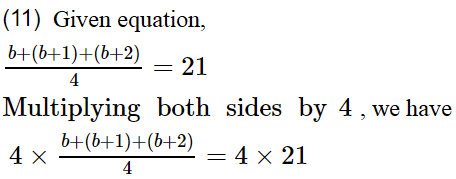b + (b + 1) + (b + 2) = 84

3b + 3 = 84

Subtracting 3 from both the sides, we have

3b + 3 – 3 = 84 – 3

3b = 81

Now, dividing by 3 both sides, we get

3b/3 = 81/3

b = 27

Thus, b = 27 is the solution of the equation.

Practice Set 12.2 Page No: 77

1. Mother is 25 year older than her son. Find son’s age if after 8 years ratio of son’s age to mother’s age will be 4/9.

Solution:

Let’s consider the present age of the son be x years.

Then, the age of the mother = (x + 25) years

Now, after 8 years

Age of son = (x + 8) years

Age of mother = (x + 25+ 8) years = (x + 33) years

From the given information, we have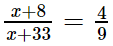On cross-multiplying,

9(x + 8) = 4(x + 33)

9x + 72 = 4x + 132

9x – 4x = 132 – 72

5x = 60

⇒ x = 12

Thus, the present age of the son is 12 years.

2. The denominator of a fraction is greater than its numerator by 12. If the numerator is decreased by 2 and the denominator is increased by 7, the new fraction is equivalent with 1/2. Find the fraction.

Solution:

Let’s assume the numerator of the fraction to be x.

So,

Denominator = x + 12

The factor = x/(x + 12)

Now, from the given information we have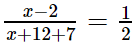On cross multiplying,

2 (x – 2) = (x + 12 + 7)

2x – 4 = x + 19

2x – x = 19 + 4

x = 23

Hence, the numerator of the fraction is 23 and the denominator of the fraction is (23 + 12) = 35.

Therefore, the fraction is 23/35.

3. The ratio of weights of copper and zinc in brass is 13:7. Find the weight of zinc in a brass utensil weighing 700 gm.

Solution:

Given that the ratio of weights of copper and zinc in brass is 13: 7.

So, let the weight of the copper in brass be 13x

And the weight of zinc in brass be 7x

Now, from the given information we have

Weight of brass = 700 gm

⇒ Weight of copper in brass + weight of zinc in brass = 700 gm

13x + 7x = 700

20x = 700

x = 700/20

x = 35

Therefore, the wight of zinc in brass = 7 x 35 = 245 gm.

4. Find three consecutive whole numbers whose sum is more than 45 but less than 54.

Solution:

Let’s take the three consecutive whole numbers to be x, x + 1 and x + 2.

The given conditions are,

45 < x + (x + 1) + (x + 2) and x + (x + 1) + (x + 2) < 54

45 < 3x + 3 and 3x + 3 < 54

45 – 3 < 3x and 3x < 54 – 3

42 < 3x and 3x < 51

14 < x and x < 17

So, x = 15, 16

Now, if x = 15

The other numbers are 16, 17.

If x = 16,

The other number are 17, 18.

Therefore, the three consecutive whole number are 15, 16, 17 or 16, 17, 18.

5. In a two-digit number, digit at the ten’s place is twice the digit at unit’s place. If the number obtained by interchanging the digits is added to the original number, the sum is 66. Find the number.

Solution:

Let’s assume the digit at the unit’s place to be x.

Digital at tens place = 2x

Original number = 2x × 10 + x = 21x

Now, the number obtained by interchanged the digits = x × 10 + 2x = 12x

From the given information,

12x + 21x = 66

33x = 66

⇒ x = 2

So, the unit’s digit = 2 and the ten’s digit = 2 x 2 = 4

Therefore, the number is 42.

6. Some tickets of Rs 200 and some of Rs 100, of a drama in a theatre were sold. The number of tickets of Rs 200 sold was 20 more than the number of tickets of Rs 100. The total amount received by the theatre by sale of tickets was Rs 37000. Find the number of Rs 100 tickets sold.

Solution:

Let’s consider the number of tickets of Rs 100 be x.

Then, the number of tickets of Rs 200 = x + 20

From the given information,

100 (x) + 200 (x + 20) = 37000

100x + 200x + 4000 = 37000

300x = 37000 – 400

300x = 33000

⇒ x = 110

The number of tickets of Rs 100 = 110.

And, the number of tickets of Rs 200 = 110 + 20 = 130.

Therefore, the number of tickets of Rs 100 is 110.

7. Of the three consecutive natural numbers, five times the smallest number is 9 more than four times the greatest number, find the numbers.

Solution:

Let’s consider the three consecutive natural numbers to be x, x + 1 and x + 2.

From the given information,

5 (x) = 4 (x + 2) + 9

5x = 4x + 8 + 9

5x – 4x = 17

⇒ x = 17

So, the three consecutive natural number are 17, 17 + 1 and 17 + 2.

Therefore, the three consecutive natural number are 17, 18 and 19.

8. Raju sold a bicycle to Amit at 8% profit. Amit repaired it spending Rs 54. Then he sold the bicycle to Nikhil for Rs 1134 with no loss and no profit. Find the cost price of the bicycle for which Raju purchased it.

Solution:

Let’s assume the cost price of the bicycle for which Raju purchased it be x.

And the selling of the bicycle = 108% of cost price

= 108/100 × x

Also given, Amit repaired the cycle spending Rs 54.

So,

The cost price of the bicycle for which Nikhil purchased = (108/100)x + 54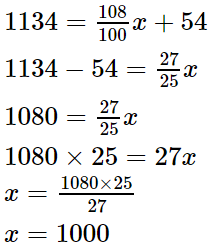x = (1080 x 25)/ 27

x = 1000

Therefore, the cost price of the bicycle for which Raju purchased it is Rs 1000.

9. A Cricket player scored 180 runs in the first match and 257 runs in the second match. Find the number of runs he should score in the third match so that the average of runs in the three matches be 230.

Solution:

Given,

Runs scored in the first match = 180

Runs scored is the second match = 257

So,

The total run scored in all three matches = Average x Number of matches

= 230 x 3

= 690

Now,

Run scored in third match = 690 – (180 + 257)

= 690 – 437

= 253

Therefore, the runs he should score in the third match is 253.

10. Sudhir’s present age is 5 more than three times the age of Viru. Anil’s age is half the age of Sudhir. If the ratio of the sum of Sudhir’s and Viru’s age to three times Anil’s age is 5:6, then find Viru’s age.

Solution:

Let’s take Viru’s present age to be ‘x’ years.

Now, Sudhir’s present age = (5 + 3x) years

Anil’s present age = (5 + 3x)/ 3 years

From the given information,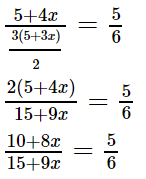6 (10 + 8x) = 5 (15 + 9x)

60 + 48x = 75 + 45x

48x – 45x = 75 – 60

3x = 15

x = 5

Therefore, Viru’s age is 5 years.

The value of the variable, which satisfies the given equation is called the solution of the equation. This chapter deals with equations in one variable only. Solving an equation means to find the solution of the equation. The equation obtained by performing the same operation on both sides does not change its solution. Using this property, we obtain new but simpler equations and solve the given equation.

## Frequently Asked Questions on Maharashtra State Board Solutions for Class 8 Maths Chapter 12 Equations in One Variable

### Can we use these Maharashtra State Board Class 8 Maths Chapter 12 Solutions to prepare for the exams?

Yes, these Maharashtra State Board Solutions for Class 8 Maths Chapter 12 Equations in One Variable is will help to prepare for the board exams. Students are highly recommended to practise these solutions after revising the subjects, as they set the basis for the questions that could get asked in the board exams.

### How can students make the best use of these solutions?

Students are encouraged to practise these questions and then to refer back to the solutions to analyse their performance. This will also help the students to rectify the mistakes and avoid making them during the board exams.

### Is is possible to download these Maharashtra State Board Class 8 Maths Chapter 12 Solutions?

If a sufficiently large quantity of solvent dissolves a small volume of solvent, the solution is considered a dilute solution. Concentrated solution is when the quantity of solvent dissolved is relatively high compared to the quantity of water, then concentrated solution is called solution.